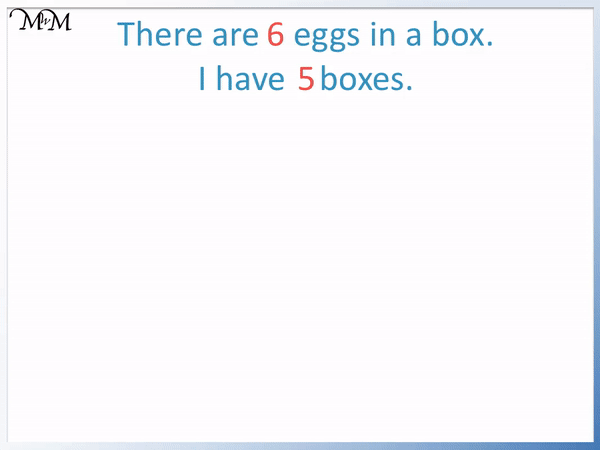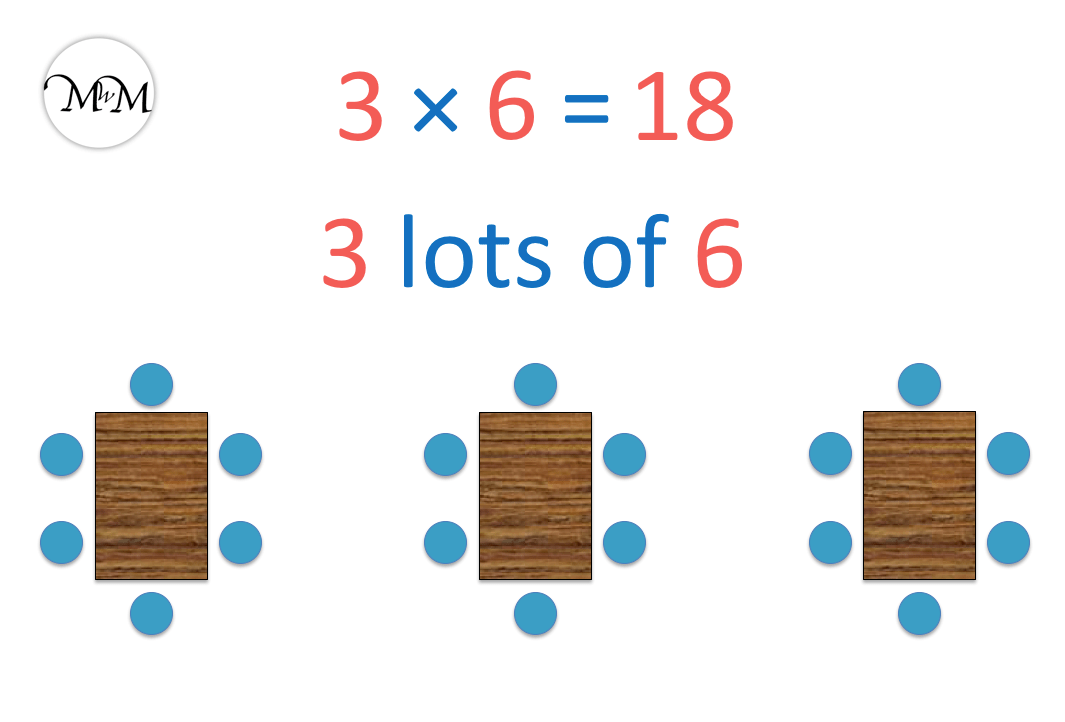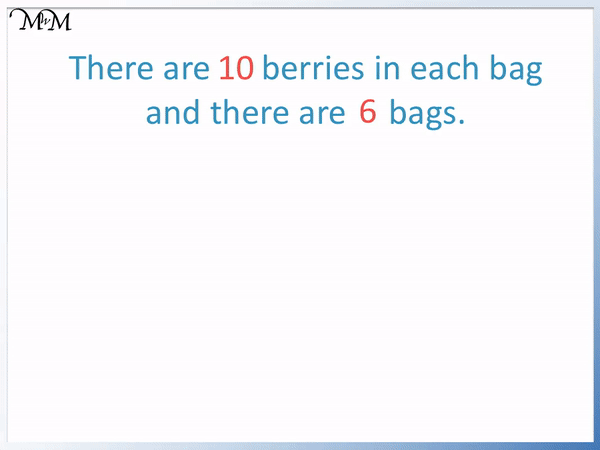# Multiplication Word Problems

Multiplication Word Problems• There are 3 tables and there are 6 children on each table.
• The children are in equal groups of 6.
• There a 3 groups.
• We have 3 lots of 6, which we can write as a multiplication.
• 3 ×6 = 18 and so we have 18 children.
• We multiplied the number of groups by the number in each group to find the total.

When we have equal groups of the same number, we multiply to find the total.• There are 6 eggs in each box and I have 5 boxes.
• I have 5 lots of 6 eggs.
• When we have the same amount in each group we have a multiplication.
• We multiply the number of groups by the amount in each group to find the total.
• We have 5 groups of 6 and 5 × 6 = 30.
• There are 30 eggs in total.Supporting Lessons# Multiplication Word Problems

## How to Identify Multiplication Word Problems

When we have equal groups of of the same amount, we can use multiplication to find the total.

The number of groups multiplied by the amount in each group gives us the total.

The best way to identify a multiplication word problem is to read the question fully and try to understand the context.

If we have multiple groups with an equal number of items in and we need a total, then we have a multiplication problem.

Keywords may help identify a multiplication. Some multiplication keywords are:

• Lots of
• Groups of
• Each
• Altogether
• Total
• In all

However, these keywords are only a clue to multiplication and we should always check the context of the question. For example, words such as ‘altogether’ and ‘in all’ may indicate addition instead.

## Simple Multiplication Word Problems

To solve multiplication word problems follow these steps:

1. Identify the numbers in the question.
2. Identify which of these numbers tells us how many there are in each group.
3. Identify which of these numbers tells us how many groups there are.
4. Multiply these two numbers using times tables or long multiplication.
5. In these examples we will look at simple multiplication word problems in which there is not a large paragraph of text and the numbers to multiply will be less than 12.

In this question ‘There are 3 tables and there are 6 children on each table.’ We are asked, ‘How many children are there in total?’

We have 6 children on each table, so there are 6 in each group.

We have 3 tables, so 3 groups of children.

We are asked for the total. To find the total, we multiply the number of groups by the amount in each group.We have 3 groups of 6, or 3 lots of 6 children.

The word ‘lots of’ can be replaced with a multiplication sign.3 × 6 = 18 and so, there are 18 children in total.

As long as we have an equal number in each group then we can multiply to see how many there are in total. We had 6 children on every table.

The words each and total together in this question could have given us a clue that we may have had a multiplication.

When teaching multiplication word problems, it is very important to understand the context. To do so, we try to imagine what is actually happening in real life.

Encourage children to draw the situation or model it using cubes or counters.

In these examples we have drawn out the situation to help visualise what is going on.

In this next example, ‘There are 6 eggs in a box. I have 5 boxes. How many eggs are there in total?’

There are 6 eggs in each group.

We have 5 boxes, so 5 lots of 6.

We have an equal number of items in each group and so, to find the total, we multiply the number of groups by the number of items in each group.We have 5 boxes of 6, or 5 lots of 6 eggs.

5 × 6 = 30 and so, there are 30 eggs in total in the 5 boxes.

We could multiply in this word problem because there were the same number of eggs in each box.

Again, when explaining these multiplication word problems, it is helpful to draw or model the situation if it is difficult to conceptualise.

Here instead of drawing egg boxes, you could draw a circle to represent each egg, or use 5 pile of 6 counters.

In this example, ‘There are 10 berries in each bag and there are 6 bags. How many berries are there in total?’

We have 10 berries in each bag.

There are 6 bags.

We have the same number of berries in each bag and so we multiply to find the total.We have 6 lots of 10.

6 × 10 = 60 and so, there are 60 berries in total.

With larger numbers, it is not a good idea to use 60 counters or draw 60 berries.

We can draw the 6 bags and label a 10 by each.

We can then count on in tens 6 times to reach our answer.

In this example, ‘Each lollipop costs $2. I buy 8 lollipops. How much do they cost in total?’ With multiplication word problems involving money, we multiply the number of items by the amount each costs. We are spending$2 for each lollipop.

We spend $2 eight times.8 ×$2 = $16 and so, the 8 lollipops cost$16.Now try our lesson on Grid Method Multiplication where we learn how to work out larger multiplications using the grid method.